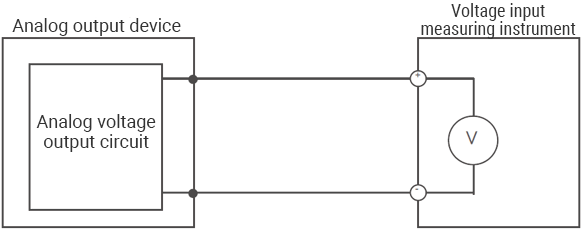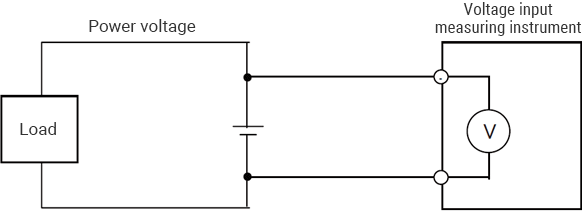# How to Measure Voltage

Voltage is measured in two ways—measuring the analog voltage output from a sensor and measuring the power voltage—each of which is explained in this section.

## Measuring the analog voltage output from a sensorThe output voltage device can be measured by inputting + and − from the target analog voltage output circuit directly into a voltage input measuring instrument.

## Measuring the power voltageThe voltage can be measured by connecting probes to the + and − terminals of the target voltage.

INDEX